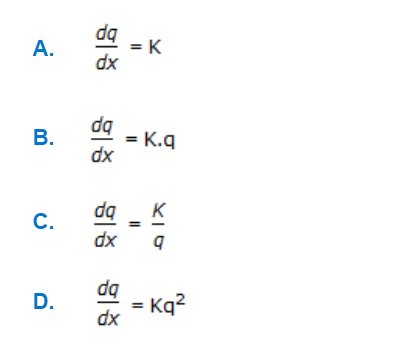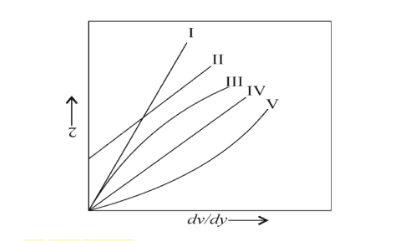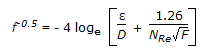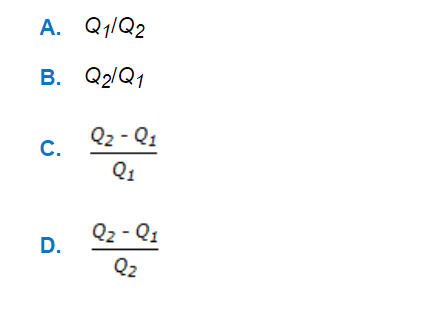# E-PolyLearning

 41. The inherent characteristic of an equal percentage valve relating flow rate 'q' with valve stem movement 'x' are described by the equationa. A b. B c. C d. D

 42. Curve III in the below diagram represents a/ana. dilatent fluid b. pseudo plastic fluid c. ideal plastic d. none of these
 43. Colebrook equation for friction factor in turbulent flow is given by following equation. It reduces to Nikuradse equation for a value of ε/D equal toa. 0 b. 1 c. ∞ d. 0.5
 44. The percentage slip in a reciprocating pump set is given by the % of (where, Q₁ = actual discharge Q₂ = theoretical discharge)a. A b. B c. C d. D